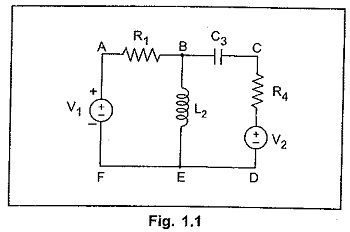## Network Terminology:

In this Network Terminology, we shall define some of the basic terms which are commonly associated with a network.

### 1.Network

Any arrangement of the various electrical energy sources along with the different circuit elements is called an electrical network. Such a network is shown in the Fig. 1.1.

### 2.Network Element

Any individual circuit element with two terminals which can be connected to other circuit element, is called a network element.

Network elements can be either active elements or passive elements. Active elements are the elements which supply power or energy to the network. Voltage source and current source are the examples of active elements. Passive elements are the elements which either store energy or dissipate energy in the form of heat. Resistor, inductor and capacitor are the three basic passive elements. Inductors and capacitors can store energy and resistors dissipate energy in the form of heat.

### 3.Branch

A part of the network which connects the various points of the network with one another is called a branch. In Fig. 1.1, A-B, B-C, C-D etc. are called the branches of the network.

### 4.Junction Point

A point where three or more branches meet is called a junction point. Point B and E are the junction points in the network shown in the Fig. 1.1.

### 5.Node

A point at which two or more elements are joined together is called node. The junction points are also the nodes of the network. In the network shown in the Fig. 1.1, A, B, C, D, E and F are the nodes of the network.

### 6.Mesh (or Loop)Mesh (or Loop) is a set of branches forming a dosed path in a network in such a way that if one branch is removed then remaining branches do not form a closed path. A loop also can be defined as a closed path which originates from a particular node, terminating at the same node, travelling through various other nodes, without travelling through any node twice. In the Fig. 1.1 paths ABEFA, ABCDEFA, BCDEB etc, are the loops of the network.

Scroll to Top